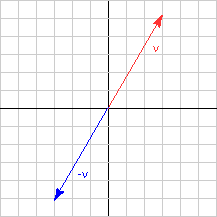What is the relation between the length of v and the length of -v?

| v | = | -v |

# Opposite DirectionYou can see this mechanically:

```| v | = |(a, b, c)T| = √( a2 + b2 + c2)

|-v | = |(-a, -b, -c)T|

=  √( -a2 + -b2 + -c2 )

=  √( a2 + b2 + c2 )
```

The diagram shows this graphically (in two dimensions).

You would like to say that the two vectors are the same length, but point in opposite directions. In fact, you can say that. The next chapter will give you the authority to do so.

### QUESTION 13:

What is the length of the vector represented by (1, -1, 1)T     ?